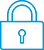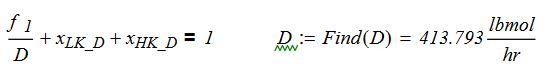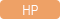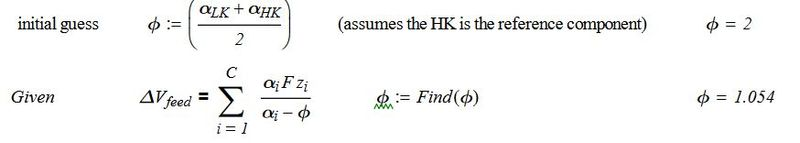cancel
Showing results for
Search instead for
Did you mean:It has been a while since anyone has replied. Simply ask a new question if you would like to start the discussion again.Level 1
10 3 0 0
Message 1 of 9
1,217
Flag Post

# Use solve function in programHP Recommended
HP Prime

Hi,

I was trying to solve the equation below using the solve command. I used the synntax solve(Expr,[Var] ) but it still did not work in my program. This equation is one of many so i am trying to make one program that solve many equation and I am stuck at this one.So It would be better for me to make a program then use the solve app.Can anyone help me to get this work?Tags (3)
8 REPLIES 8Level 6
252 251 42 77
Message 2 of 9
Flag PostHP Recommended

Hello,

Are you trying to do a symbolic or numerical solve?

If symbolic, then you need to make sure that you use the CAS version.

If numerical, remember that the solve function is a function aplet function (yes, seems confusing I know :-)). by this I mean that either the funciton app needs to be the current one, or you need to call Function.Solve...

I do not remember if the numerical solve function works with program local variables. This might be the problem.

Cyrille

I am an HP EmployeeLevel 1
10 3 0 0
Message 3 of 9
Flag PostHP Recommended

Cyrille,

Thanks for the response. It is numerical.  Here is a sample code I have, I tried to add Function.solve and have the  Function app as the app open but it still gives me syntax error.

Is it not possible to use to do this kind of numerical operation in a program on the hp prime?

```EXPORT Prog(X_lkd, X_hkd,F)
BEGIN
solve(F/D+X_lkd+X_hkd=1,D)RETURN D
END; H```Level 6
252 251 42 77
Message 4 of 9
Flag PostHP Recommended

Hello,

The following does seem to work.

EXPORT V1, V2, V3;
EXPORT Prog(X_lkd, X_hkd,F)
BEGIN
V1:= X_lkd; V2:= X_hkd; V3:= F;
Solve.SOLVE(V3/D+V1+V2=1, D, -2);
RETURN D;
END;

If, depending on the 3 parameter, the right part of the function is always greater than 1, then the solver seems to have issues finding the root and needs a guess to work with...

Cyrille

I am an HP EmployeeLevel 1
10 3 0 0
Message 5 of 9
Flag PostHP Recommended

When I enter

X_lk = .97,X_hl=.001 ,f=12 it gives me 0.  Even when I change the intial guess to 413 it still gives me 0.The correct answer should be what I post ealier around 413.79 is there a way to get it to work with number above 0?Level 6
252 251 42 77
Message 6 of 9
Flag PostHP Recommended

Hello,

Changing the equation do V3+D(V1+V2)=D makes it much easier to solve for the system and reduces the issues!

Having made the change, things seems to work much much better...

Cyrille

I am an HP EmployeeLevel 1
10 3 0 0
Message 7 of 9
Flag PostHP Recommended

Cyrille,

one last thing I would like to add to my program that i can not figure out. I would like to find phi such that it is in between these paremeters.

The probelm I have isThe probelma are

1) The index number can change. I would like for the program to ask for the index number so i can enter in the correcet parameters. for example if i=3 then I would like for the program to know I need 3 alphas and 3 Z and the user can enter the values.

2) I do not know how to code to solve for phi  inside the sumation.

I would like to find phi such that it is in between these paremeters.Level 6
305 297 32 76
Message 8 of 9
Flag PostHP Recommended

@Unluckyowl1wrote:

When I enter

X_lk = .97,X_hl=.001 ,f=12 it gives me 0.  Even when I change the intial guess to 413 it still gives me 0.The correct answer should be what I post ealier around 413.79 is there a way to get it to work with number above 0?

The reason is that SOLVE does not change D, it just returns a number.

You ask to return D, which will be the same as it was before running he program.

If you want to change D write:

D:=Solve.SOLVE.....

RETURN D;

Or just write:

RETURN Solve.SOLVE.....Level 6
305 297 32 76
Message 9 of 9
Flag PostHP Recommended

@Unluckyowl1wrote:

...........

Is it not possible to use to do this kind of numerical operation in a program on the hp prime?

```EXPORT Prog(X_lkd, X_hkd,F)
BEGIN
solve(F/D+X_lkd+X_hkd=1,D)RETURN D
END; H```

Suppose you want to solve SIN(10*D)+D/10+X_lkd+X_hkd=0 between the lower limit: a and the upper limit: b.

Then you could write this CAS program:

```#cas
cas_myprog1(X_lkd,X_hkd,a,b):=
BEGIN
RETURN solve(SIN(10*D)+D/10+X_lkd+X_hkd=0,D,a..b);
END;
#end```

Or alternatively:

```#cas
cas_myprog1(X_lkd,X_hkd,a,b):=
BEGIN
RETURN solve(SIN(10*D)+D/10+X_lkd+X_hkd=0,D=a..b);
END;
#end```

You can find the solve and fsolve commands and their Help via:

Toolbox - CAS - Solve - (Solve or  Numerical Solve)

The differences between solve and fsolve are not very big, but solve is primarily meant for exact calculations and fsolve for numerical ones.

Because solve and fsolve are CAS commands it is easiest to use them in a CAS program as we did here.

It is also possible to use them in a non-CAS program with the construction CAS("....").

Then we get:

```EXPORT MYPROG1(X_lkd,X_hkd,a,b)
BEGIN
RETURN CAS("solve(SIN(10*D)+D/10+X_lkd+X_hkd=0,D,a..b)");
END;```

Or:

```EXPORT MYPROG1(X_lkd,X_hkd,a,b)
BEGIN
LOCAL s;
s:="solve(SIN(10*D)+D/10+X_lkd+X_hkd=0,D,a..b)";
RETURN CAS(EVAL(s));
END;```

Still another way is to use this construction:

```EXPORT MYPROG1(X_lkd,X_hkd,a,b)
BEGIN
RETURN CAS.solve("SIN(10*D)+D/10+X_lkd+X_hkd=0","D","a..b");
END;```

So solve is not the same as the app command SOLVE.

In fact you can choose between:

-The strong exact solver: solve.

-The strong numerical solver: fsolve

-The Home solver: FNROOT

-The Function app solver: ROOT

-The Solve app solver: SOLVE.

See also this article for more inormation:

http://www.hpmuseum.org/forum/thread-3149.html

† The opinions expressed above are the personal opinions of the authors, not of HP. By using this site, you accept the Terms of Use and Rules of Participation